Time Domain Specifications

# Time Domain Specifications | Control Systems - Electrical Engineering (EE)

 1 Crore+ students have signed up on EduRev. Have you?

In this chapter, let us discuss the time domain specifications of the second order system. The step response of the second order system for the underdamped case is shown in the following figure.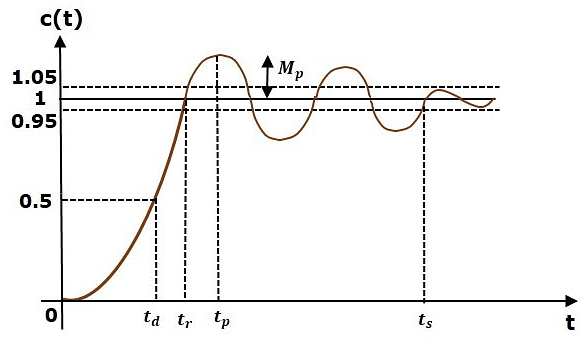All the time domain specifications are represented in this figure. The response up to the settling time is known as transient response and the response after the settling time is known as steady state response.

Delay Time

It is the time required for the response to reach half of its final value from the zero instant. It is denoted by td.
Consider the step response of the second order system for t ≥ 0, when ‘δ’ lies between zero and one.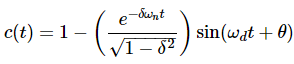The final value of the step response is one.

Therefore, at t=td, the value of the step response will be 0.5. Substitute, these values in the above equation.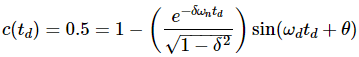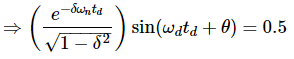By using linear approximation, you will get the delay time td as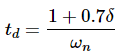Rise Time

It is the time required for the response to rise from 0% to 100% of its final value. This is applicable for the under-damped systems. For the over-damped systems, consider the duration from 10% to 90% of the final value. Rise time is denoted by tr.
At t = t1 = 0, c(t) = 0.
We know that the final value of the step response is one.
Therefore, at t = t2, the value of step response is one. Substitute, these values in the following equation.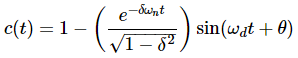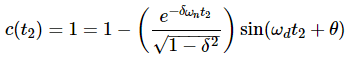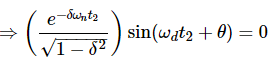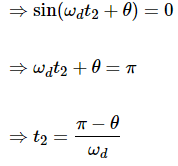Substitute t1 and t2 values in the following equation of rise time,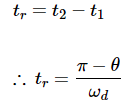From above equation, we can conclude that the rise time tr and the damped frequency ωd are inversely proportional to each other.

Peak Time

It is the time required for the response to reach the peak value for the first time. It is denoted by tp. At t=tp, the first derivate of the response is zero.
We know the step response of second order system for under-damped case is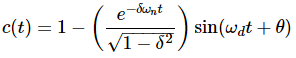Differentiate c(t) with respect to ‘t’.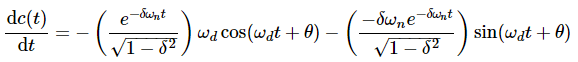Substitute, t=tp and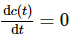in the above equation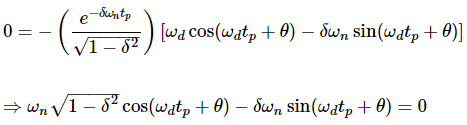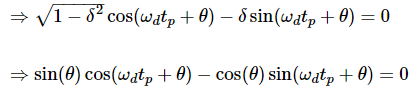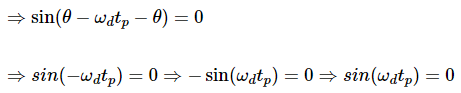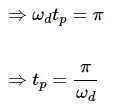From the above equation, we can conclude that the peak time tp and the damped frequency ωd are inversely proportional to each other.

Peak Overshoot
Peak overshoot Mp is defined as the deviation of the response at peak time from the final value of response. It is also called the maximum overshoot.
Mathematically, we can write it as
Mp=c(tp)−c(∞)
Where,
c(tp) is the peak value of the response.
c(∞) is the final (steady state) value of the response.
At t=tp, the response c(t) is -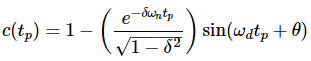Substitute,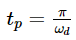in the right hand side of the above equation.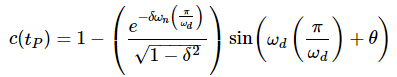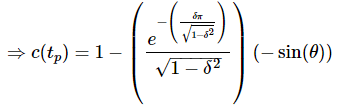We know that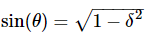So, we will get c(tp) as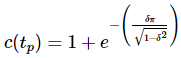Substitute the values of c(tp) and c(∞) in the peak overshoot equation.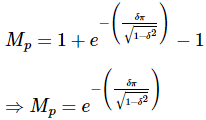Percentage of peak overshoot % Mp can be calculated by using this formula.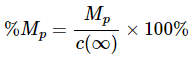By substituting the values of Mp and c(∞) in above formula, we will get the Percentage of the peak overshoot %Mp as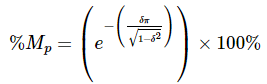From the above equation, we can conclude that the percentage of peak overshoot %Mp will decrease if the damping ratio δ increases.

Settling time

It is the time required for the response to reach the steady state and stay within the specified tolerance bands around the final value. In general, the tolerance bands are 2% and 5%. The settling time is denoted by ts.

The settling time for 5% tolerance band is -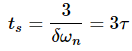The settling time for 2% tolerance band is -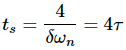Where, τ is the time constant and is equal to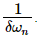• Both the settling time ts and the time constant τ are inversely proportional to the damping ratio δ.
• Both the settling time ts and the time constant τ are independent of the system gain. That means even the system gain changes, the settling time ts and time constant τ will never change.

Example

Let us now find the time domain specifications of a control system having the closed loop transfer function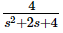when the unit step signal is applied as an input to this control system.

We know that the standard form of the transfer function of the second order closed loop control system as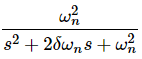By equating these two transfer functions, we will get the un-damped natural frequency ωn as 2 rad/sec and the damping ratio δ as 0.5.

We know the formula for damped frequency ωd as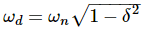Substitute, ωn and δ values in the above formula.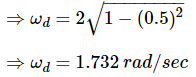Substitute, δ value in following relation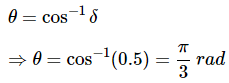Substitute the above necessary values in the formula of each time domain specification and simplify in order to get the values of time domain specifications for given transfer function.

The following table shows the formulae of time domain specifications, substitution of necessary values and the final values.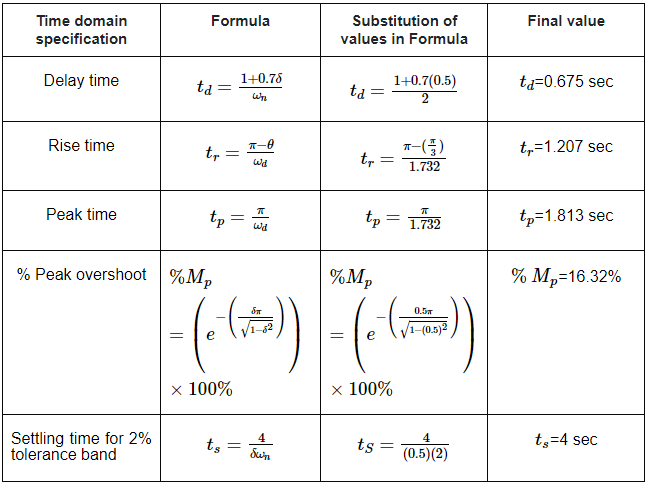The document Time Domain Specifications | Control Systems - Electrical Engineering (EE) is a part of the Electrical Engineering (EE) Course Control Systems.
All you need of Electrical Engineering (EE) at this link: Electrical Engineering (EE)

54 docs|45 tests

## Control Systems

54 docs|45 tests

Track your progress, build streaks, highlight & save important lessons and more!(Scan QR code)

,

,

,

,

,

,

,

,

,

,

,

,

,

,

,

,

,

,

,

,

,

;